# Gpu_mandelbrot

Interactive Mandelbrot set on GPU with Python
Alternatives To Gpu_mandelbrot
Deep Learning From Scratch 3426
2 years ago5mitPython
『ゼロから作る Deep Learning ❸』(O'Reilly Japan, 2020)
Gmmn132
7 years agoPython
Generative moment matching networks
Neural Gpu89
5 years ago1Python
Code for the Neural GPU model originally described in "Neural GPUs Learn Algorithms"
Gpugraphtx73
3 years ago2mitPython
Simple moving graph of GPU activity for the Jetson TX1 and Jetson TX2
Neural Network Sandbox64
a year ago13gpl-2.0Python
This is a source code of Software called Neural Network Sandbox.Neural Network Sandbox makes making and training basic feed forward neural networks easy.
Image Classifier53
5 years agomitJupyter Notebook
Final Project of the Udacity AI Programming with Python Nanodegree
Gpugraph23
4 years ago1mitPython
Simple moving graph of GPU activity for the Jetson Nano Developer Kit
Anpr Tensorflow20
4 years ago5Python
Using neural networks to build an automatic number plate recognition system.
Gpu_mandelbrot15
a year agomitPython
Interactive Mandelbrot set on GPU with Python
Autoencoder8
6 years agomitPython
Autoencoder using Theano library
Alternatives To Gpu_mandelbrot
Select To Compare

Alternative Project Comparisons

# Fast Mandelbrot set explorer

## Features

• Accelerated on GPU and CPU using numba CUDA JIT
• Interactive exploration using Matplotlib
• Use mousewheel or left/right click to zoom in/out
• Use sliders to change the rendering parameters
• Save still and animated images (GIF)
• Smooth iteration coloring, anti-aliasing by oversampling
• Customizable color palette
• Precision: zoom down to float64 precision (approx. 16 significants digits)
• 100% Python

## Quick start

``````from mandelbrot import Mandelbrot
mand = Mandelbrot() # set `gpu = False` if no GPU is available
``````

### Explore the set

``````# Explore the set using interactive Matplotlib GUI
mand.explore()
``````

### Draw an image

``````# Draw an image and save it to file
mand.draw('mandelbrot.png')
``````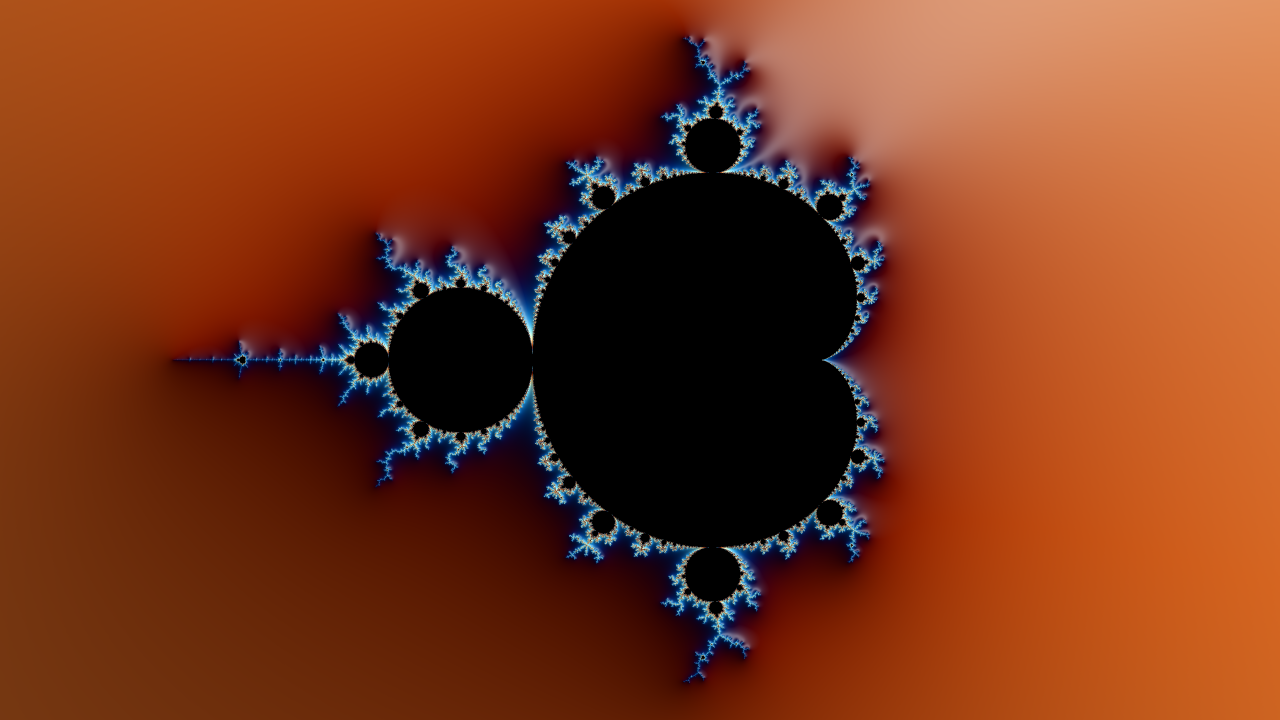### Make a zoom animation

``````# We decrease the image size to avoid overloading git and the readme page
# We also increase the number of iterations, and add stripe coloring
mand = Mandelbrot(maxiter = 5000, xpixels = 426, stripe_s = 5)
# Point to zoom at
x_real = -1.749705768080503
x_imag = -6.13369029080495e-05
mand.animate(x_real, x_imag, 'mandelbrot.gif')
``````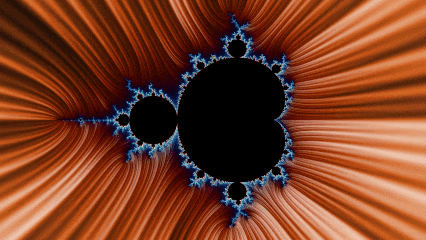### Run from command line

Use the following command from terminal to launch a GUI with default parameters:

``````python ./mandelbrot.py
``````

## Gallery

Some examples of HD images, and corresponding code:

``````mand = Mandelbrot(maxiter = 5000, rgb_thetas = [.11, .02, .92], stripe_s = 2,
coord = [-0.5503295086752807,
-0.5503293049351449,
-0.6259346555912755,
-0.625934541001796])
mand.draw('crown.png')
``````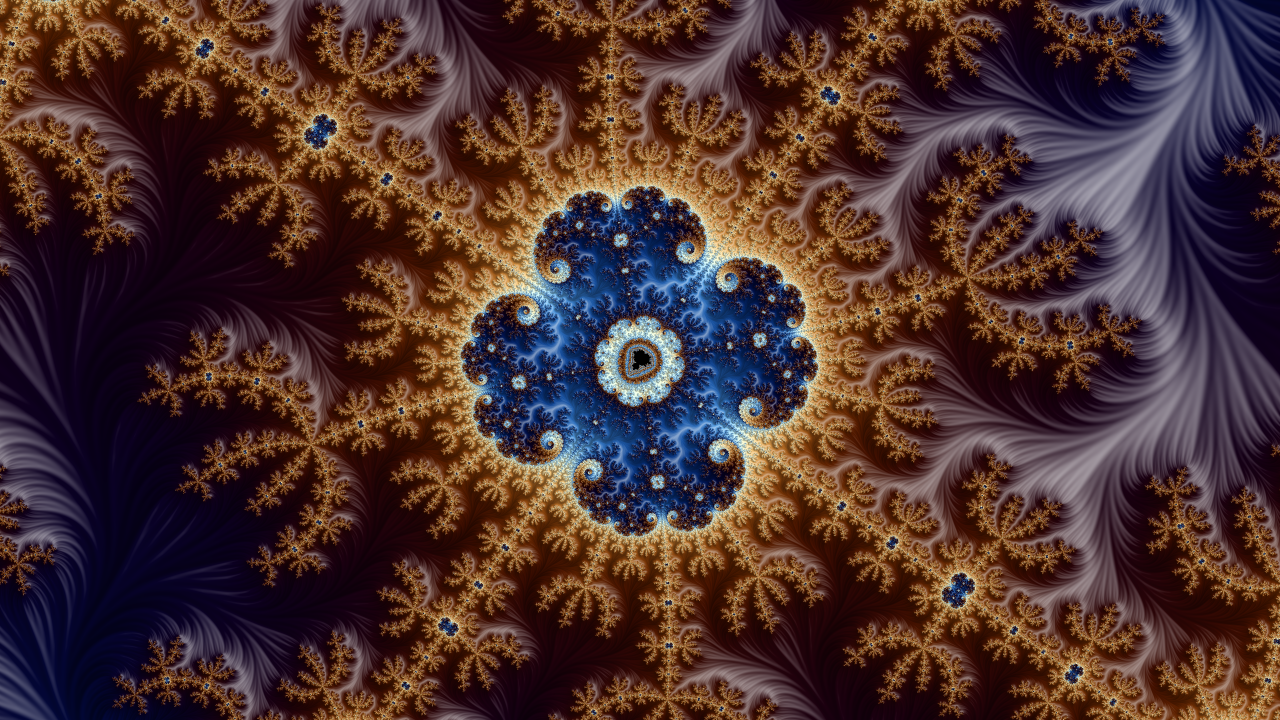``````mand = Mandelbrot(maxiter = 5000, rgb_thetas = [.29, .02, 0.9], ncycle = 8,
step_s = 10,
coord = [-1.9854527029227764,
-1.9854527027615938,
0.00019009159314173224,
0.00019009168379912058])
mand.draw('pow.png')
``````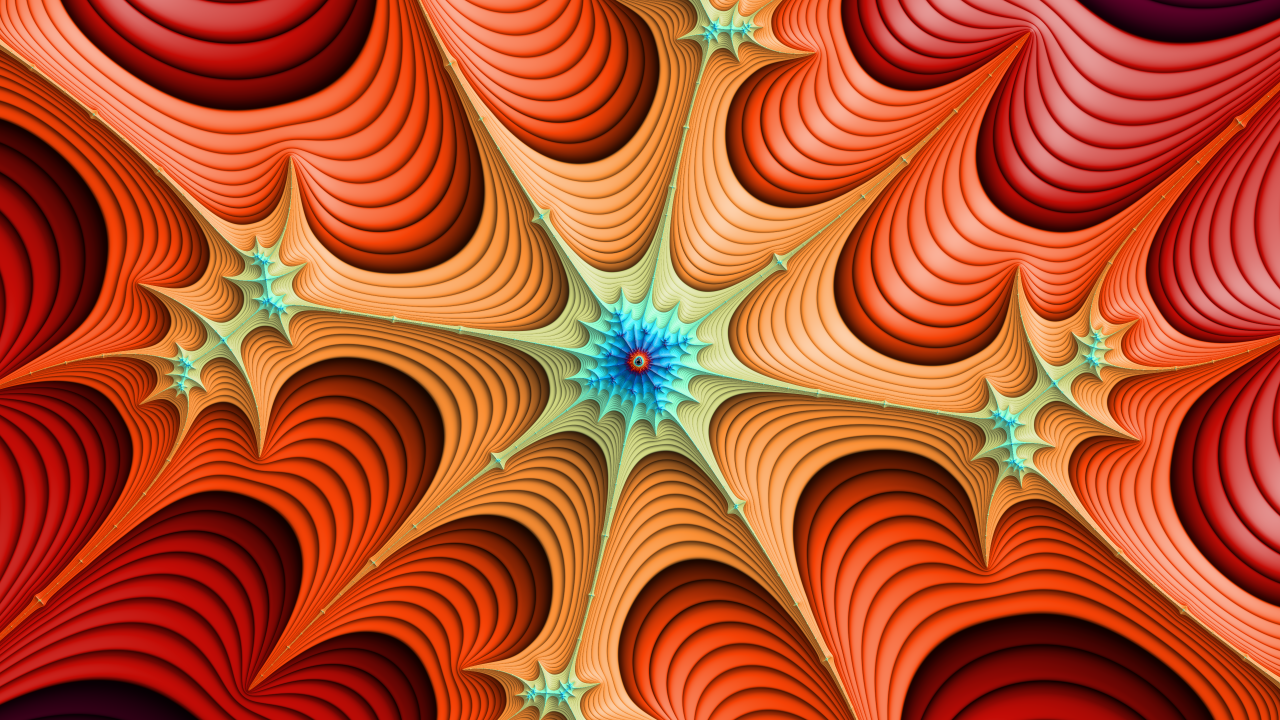``````mand = Mandelbrot(maxiter = 5000, rgb_thetas = [.83, .01, .99], stripe_s = 5,
coord = [-1.749289287806423,
-1.7492892878054118,
-1.8709586016347623e-06,
-1.8709580332005737e-06])
mand.draw('octogone.png')
``````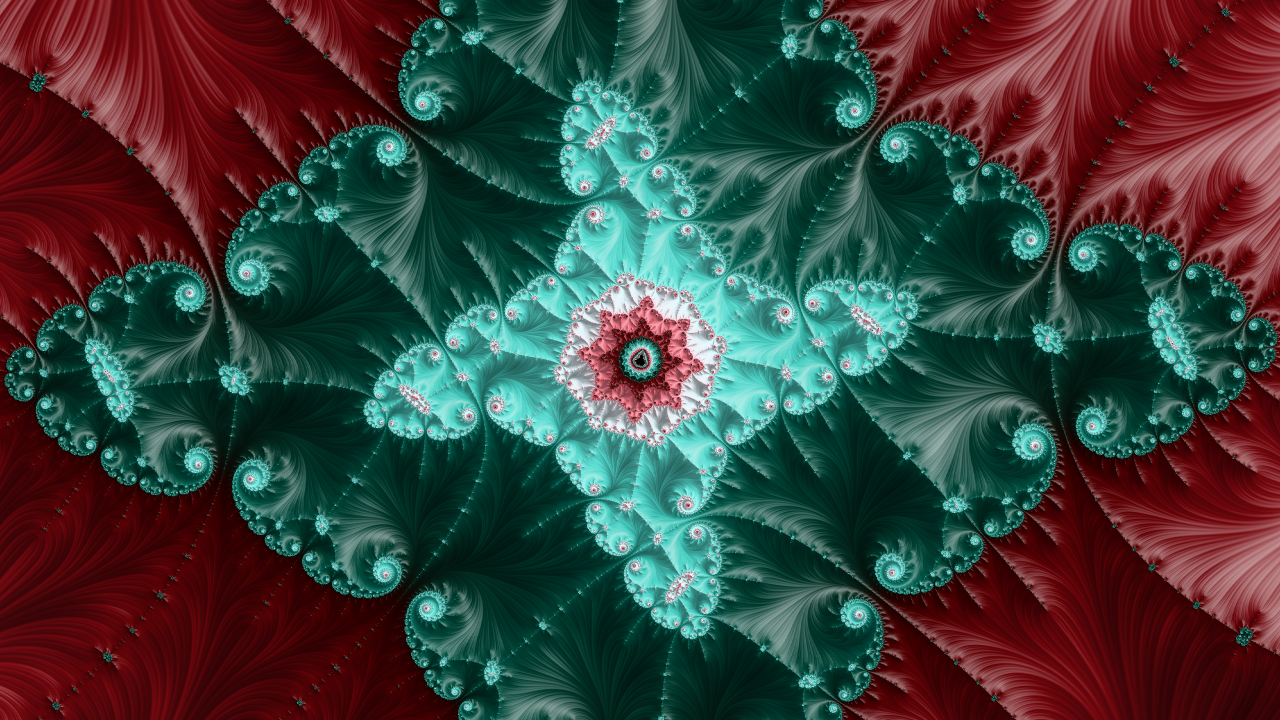``````mand = Mandelbrot(maxiter = 5000, rgb_thetas = [.87, .83, .77],
coord = [-1.9415524417847085,
-1.9415524394561112,
0.00013385928801614168,
0.00013386059768851223])
mand.draw('julia.png')
``````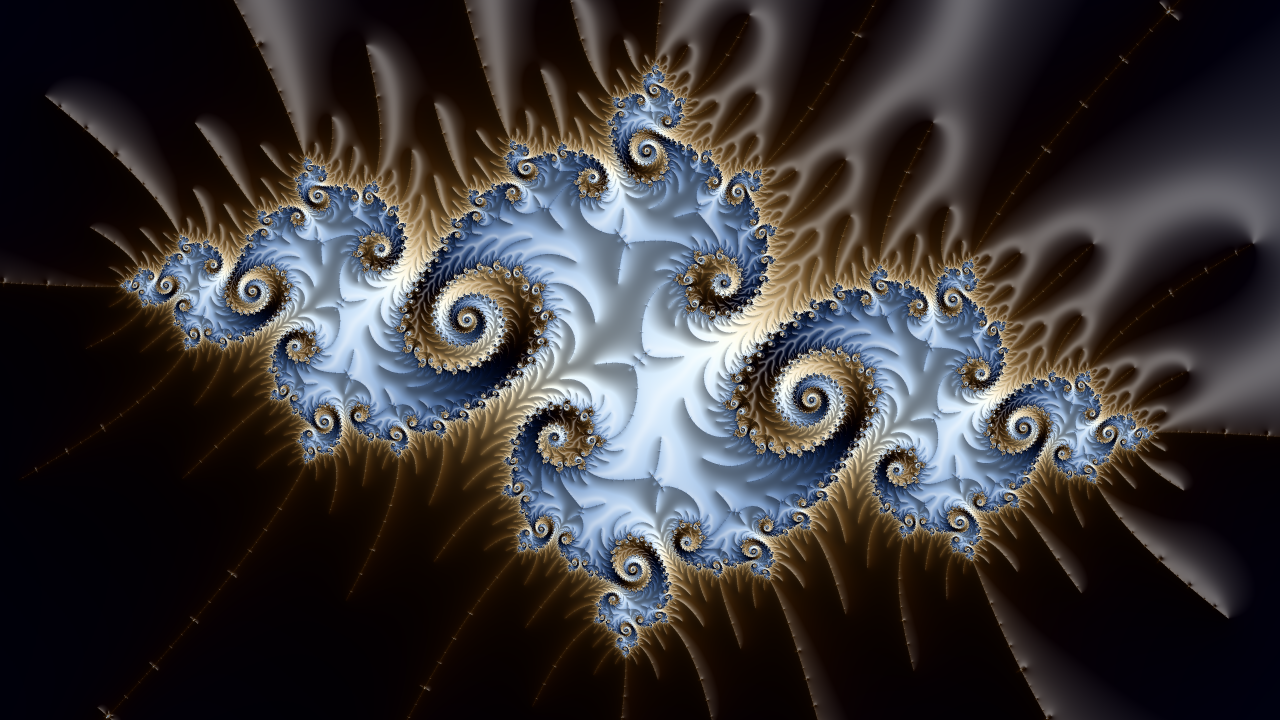``````mand = Mandelbrot(maxiter = 5000, rgb_thetas = [.54, .38, .35], stripe_s = 8,
coord = [-0.19569582393630502,
-0.19569331188751315,
1.1000276413181806,
1.10002905416902])
mand.draw('lightning.png')
``````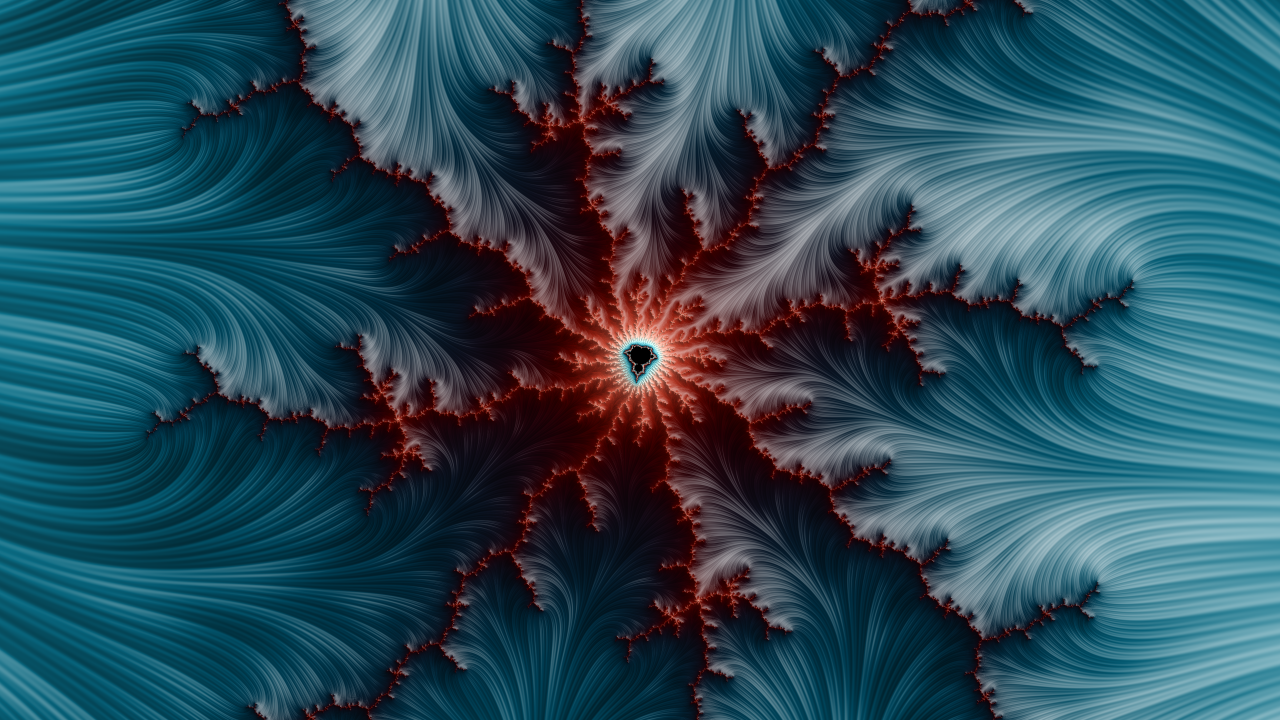``````mand = Mandelbrot(maxiter = 5000, rgb_thetas = [.47, .51, .63], step_s = 20,
coord = [-1.7497082019887222,
-1.749708201971718,
-1.3693697170765535e-07,
-1.369274301311596e-07])
mand.draw('web.png')
``````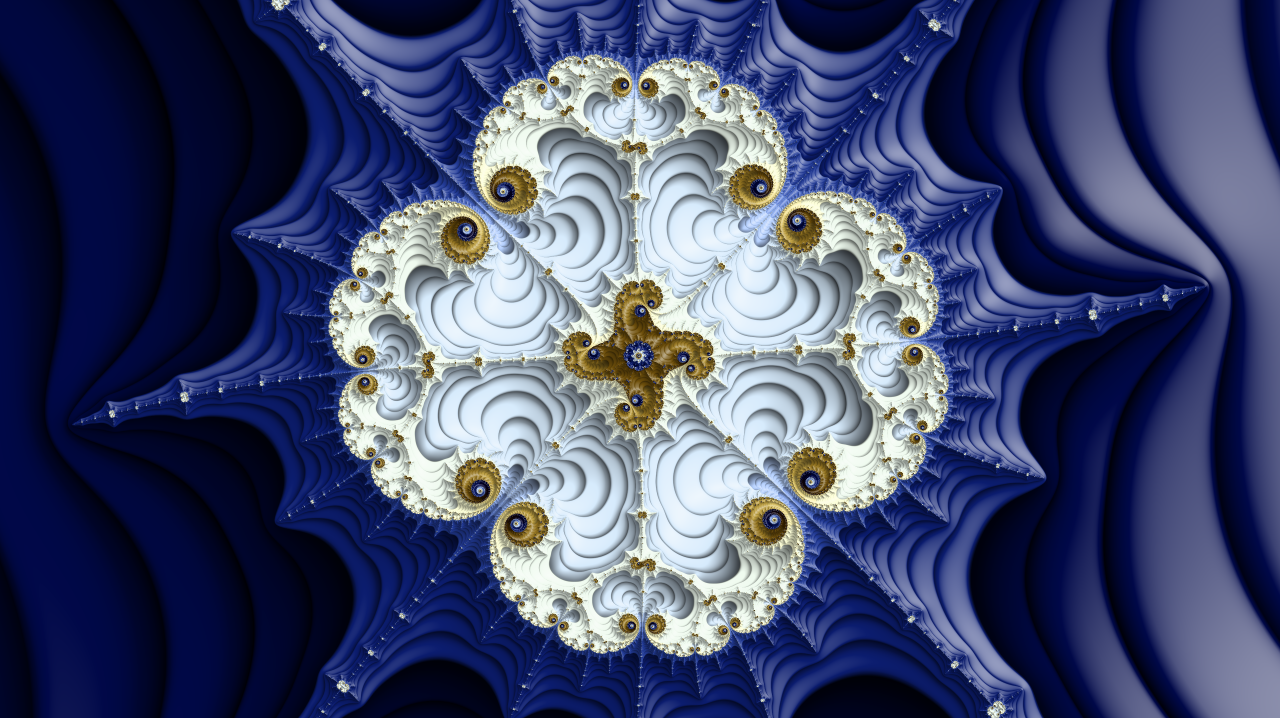``````mand = Mandelbrot(maxiter = 5000, rgb_thetas = [.6, .57, .45], stripe_s = 12,
coord = [-1.8605721473418524,
-1.860572147340747,
-3.1800170324714687e-06,
-3.180016406837821e-06])
mand.draw('wave.png')
``````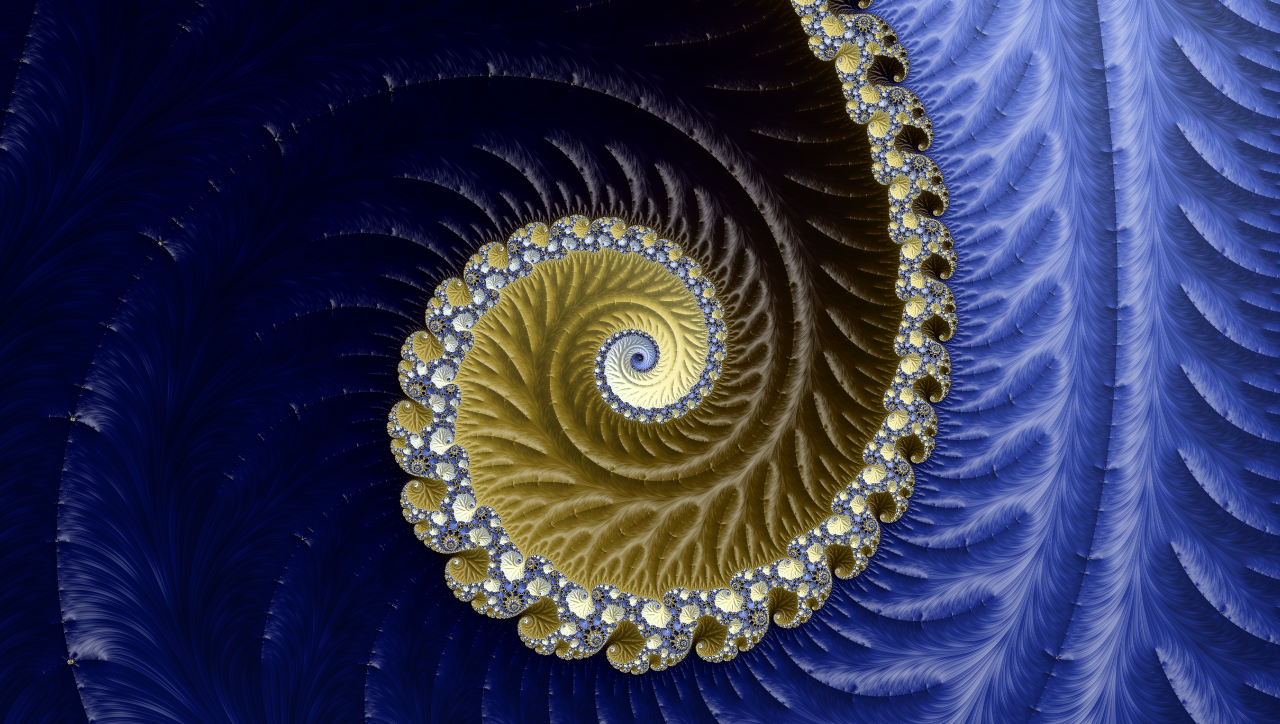``````mand = Mandelbrot(maxiter = 5000, rgb_thetas = [.63, .83, .98],
coord = [-0.7545217835886875,
-0.7544770820676441,
0.05716740181493137,
0.05719254327783547])
mand.draw('tiles.png')
``````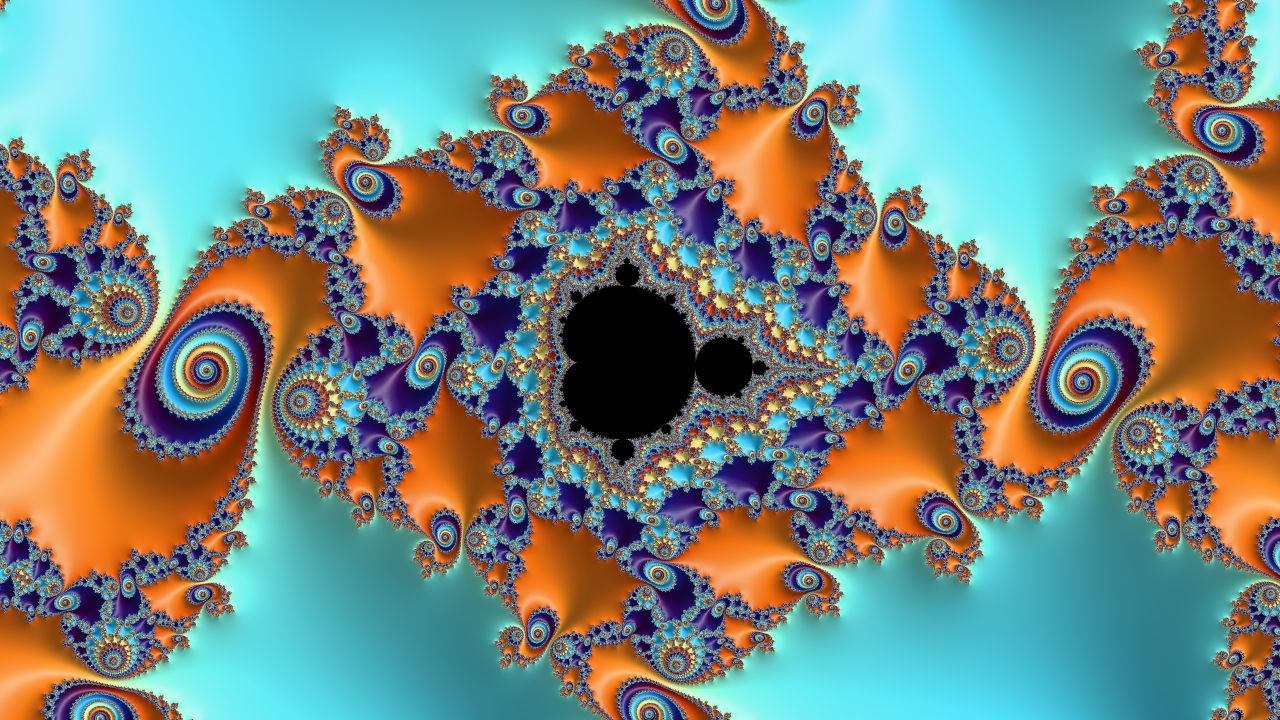``````mand = Mandelbrot(maxiter = 5000, rgb_thetas = [.29, .52, .59], stripe_s = 5,
coord = [-1.6241199193994318,
-1.624119919281773,
-0.00013088931048083944,
-0.0001308892443058033])
mand.draw('velvet.png')
``````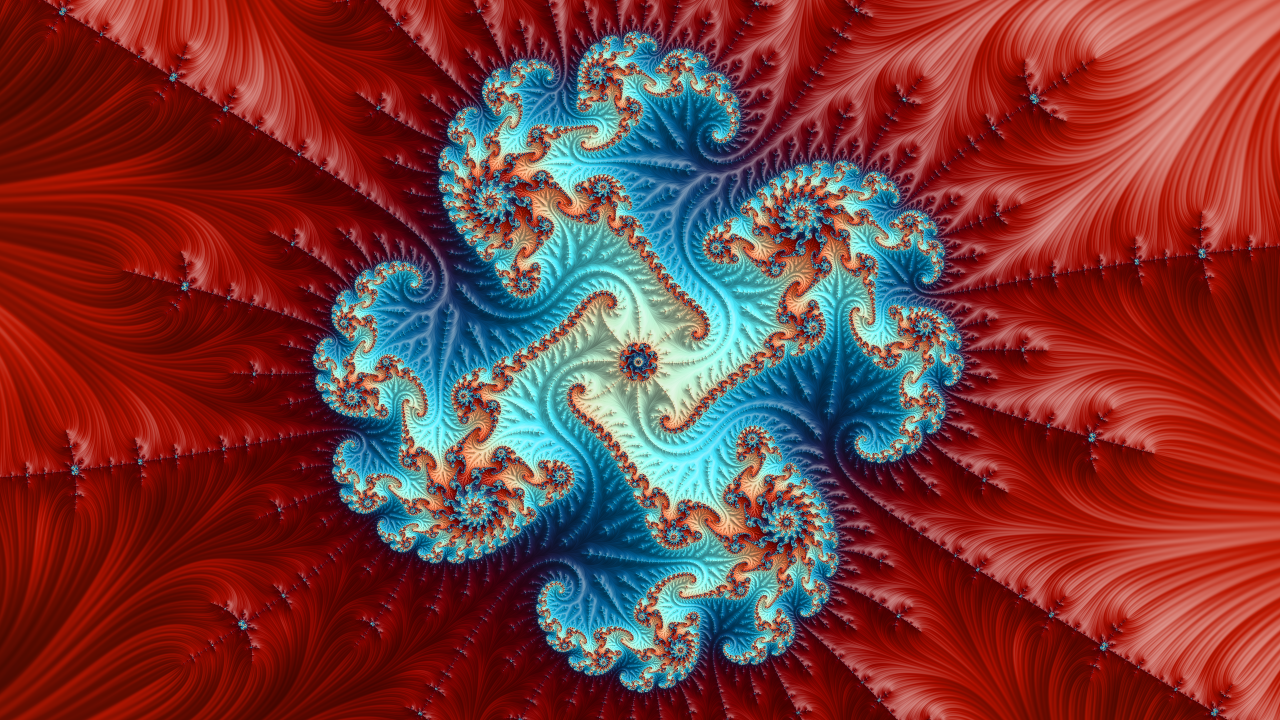## Runtime

Computing a sequence of `100` frames of HD pictures (`1280*720` pixels), with `2000` iterations takes approximately 1 second on a Tesla K80 GPU.

## Requirements

• NumPy
• Matplotlib
• Numba
• (optional, for much faster rendering) A CUDA compatible GPU & CUDA Toolkit
Popular Gpu Projects
Popular Matplotlib Projects
Popular Hardware Categories
Related Searches

Get A Weekly Email With Trending Projects For These Categories
No Spam. Unsubscribe easily at any time.
Python
Gpu
Matplotlib
Fractal